Electron. J. Differential Equations, Vol. 2017 (2017), No. 284, pp. 1-8.

### Regularity lifting result for an integral system involving Riesz potentials Yayun Li, Deyun Xu

Abstract:
In this article, we study the integral system involving the Riesz potentials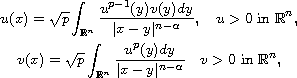where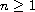,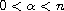and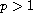. Such a system is related to the study of a static Hartree equation and the Hardy-Littlewood-Sobolev inequality. We investigate the regularity of positive solutions and prove that some integrable solutions belong to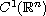. An essential regularity lifting lemma comes into play, which was established by Chen, Li and Ma .

Submitted May 16, 2017. Published November 14, 2017.
Math Subject Classifications: 35J10, 35Q55, 45E10, 45G05.
Key Words: Riesz potential; integral system; regularity lifting lemma; Hartree equation; Hardy-Littlewood-Sobolev inequality.

Show me the PDF file (218 KB), TEX file for this article.Yayun Li Institute of Mathematics School of Mathematical Sciences Nanjing Normal University Nanjing, 210023, China email: liyayun.njnu@qq.com Deyun Xu Institute of Mathematics School of Mathematical Sciences Nanjing Normal University Nanjing, 210023, China email: 954816700@qq.com